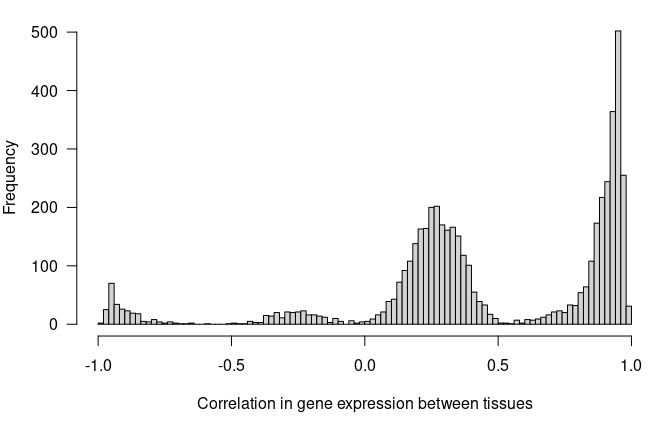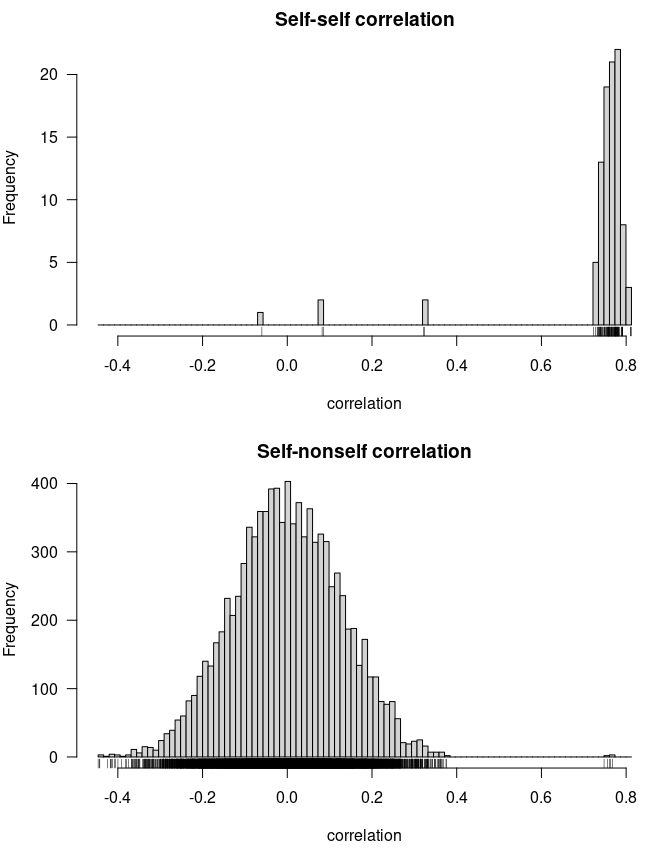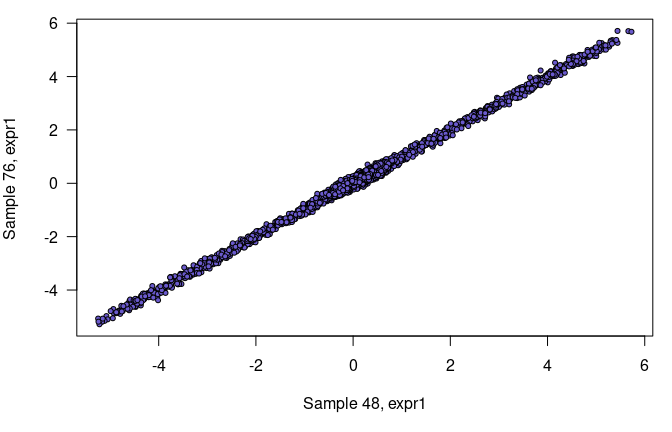# R/lineup user guide

R/lineup is an R package with tools for detecting and correcting sample mix-ups between two sets of measurements, such as between gene expression data on two tissues, and between gene expression and marker genotype data in an experimental cross. The package is particularly aimed at eQTL data for an experimental cross and as a companion to R/qtl.

This document provides a brief tutorial on the use of the package.

## Example data

We will consider a set of simulated data as an example. This is an F2 intercross with 100 individuals genotyped at 1000 autosomal markers, and with gene expression data on 5000 genes in two tissues.

We first load the R/qtl and R/lineup packages.

library(qtl)
library(lineup)

The data are immediately available, but we can also make copies in our workspace with data().

data(f2cross)
data(expr1)
data(expr2)
data(pmap)
data(genepos)

The data set f2cross is the experimental cross, in the form used by R/qtl (that is, an object of class "cross"). The data sets expr1 and expr2 are matrices with the gene expression data, with individuals as rows and genes as columns. The object pmap is a physical map of the markers in f2cross (with positions in Mbp), and genepos is a data frame with the genomic positions (in Mbp) of the genes in expr1 and expr2.

The expression data sets were stored as integers; let’s divide all values by 1000, to simplify some later plots.

expr1 <- expr1/1000
expr2 <- expr2/1000

## Lining up expression data

We’ll first consider the gene expression data in expr1 and expr2 and look for possible sample mix-ups. The basic scheme (see Broman et al. 2014) is to identify a set of genes with highly correlated expression between the two tissues, and then use these genes to measure the association between each sample in the first tissue with each sample in the second tissue.

To start, note that there are 98 individuals in each data set; there are two individuals missing from each.

nrow(expr1)
##  98
nrow(expr2)
##  98

The function findCommonID() helps to find individuals that are in common between the two data sets. For matrices, the default is to use the row names as identifiers.

eid <- findCommonID(expr1, expr2)
length(eid$first) ##  96 In the returned object, eid$first and eid$second contain indices for expr1 and expr2, respectively, to get them to line up (and omitting individuals that appear in one data set but not the other). Now let’s look at the correlation between tissues for each gene, to identify genes that are highly correlated between the two tissues. We subset the rows with the IDs in eid, so that the rows correspond (except perhaps for sample mix-ups, but we’ve not identified those yet). The function corbetw2mat() can be used to calculate portions of the correlations between columns of two different matrices . With what="paired" (the default), we assume that the two matrices have the same number of columns (say p), and that column i in the first matrix corresponds to column i in the second matrix, and we calculate just the p correlation values, for the paired columns. cor_ee <- corbetw2mat(expr1[eid$first,], expr2[eid$second,], what="paired") Here’s a histogram of these 5000 correlations. par(mar=c(5,4,1,1)) hist(cor_ee, breaks=seq(-1, 1, len=101), main="", las=1, xlab="Correlation in gene expression between tissues")You can see that this is totally contrived data. Most genes have a positive correlation between the two tissues, with a bunch in the 0 – 0.5 range, and then a bunch more near 1. But then 10% of genes are negatively correlated, again with the pattern that a bunch are in the range -0.5 – 0 and then a bunch more are near -1. Let’s focus on genes that have between-tissue correlation > 0.9 in absolute value (of which there are 1553), and then look at the correlation, across these genes, between samples in tissue 1 and samples in tissue 2. This is done with the distee() function (“dist” for distance and “ee” for expression vs. expression). d_ee <- distee(expr1[,abs(cor_ee)>0.9], expr2[,abs(cor_ee)>0.9], d.method="cor") The result is an object of class "lineupdist". If you plot the result, you’ll get two histograms: one of the self-self correlations, and another of the self-nonself correlations. par(mar=c(5,4,2,1)) plot(d_ee)For most individuals, the self-self correlation (top panel) is near 1, but there are 5 individuals with self-self correlation < 0.5. Similarly, most of the self-nonself correlations (bottom panel) are between -0.5 and 0.5, but there’s a group of 5 correlations where the self-nonself correlation is near 1. You can use the helper functions pulldiag() and omitdiag() to do these sorts of counts: pulldiag() pulls out the “diagonal” of the correlation matrix (the self-self cases), and omitdiag() sets those diagonal values to NA. So to count the number of self-self correlations that are < 0.5, we do the following. sum(pulldiag(d_ee) < 0.5) ##  5 To count the number of self-nonself correlations that are > 0.5, we do the following. d_ee_nodiag <- omitdiag(d_ee) sum( !is.na(d_ee_nodiag) & d_ee_nodiag > 0.5) ##  5 Applying the summary() function to the output of distee() gives a pair of tables that indicate potential mix-ups. summary(d_ee) ## By e1: ## maxc nextc selfc mean sd best ## 44 0.7679 0.3236 0.32180 0.0046647 0.1464 66 ## 66 0.7629 0.3608 0.32426 0.0065004 0.1517 44 ## 92 0.7607 0.3241 0.08557 -0.0092494 0.1573 24 ## 24 0.7556 0.2953 0.08221 0.0103785 0.1414 92 ## 48 0.7478 0.2466 -0.05994 0.0004805 0.1333 76 ## 52 0.3051 0.2994 NA -0.0017188 0.1284 42 ## 64 0.2574 0.2439 NA 0.0155283 0.1256 44 ## ## By e2: ## maxc nextc selfc mean sd best ## 66 0.7679 0.3262 0.32426 0.006623 0.1477 44 ## 44 0.7629 0.3577 0.32180 0.007721 0.1518 66 ## 24 0.7607 0.3270 0.08221 -0.011855 0.1576 92 ## 92 0.7556 0.2939 0.08557 0.013680 0.1417 24 ## 49 0.3379 0.3057 NA 0.016378 0.1551 16 ## 36 0.3194 0.2629 NA 0.013336 0.1144 1 ## 48 0.2231 0.2192 -0.05994 -0.014943 0.1000 71 In the first table, each row is a sample from the first data set. The first column (maxc) is the maximum correlation between that sample and the different samples in the second data set, the second column (nextc) is the next-highest correlation, and the third column (selfc) is the self-self correlation. For the rows where selfc is low but maxc is high, a sample mix-up is indicated. The last column is the sample in the second data set that has the highest correlation. The rows are ordered by the value in maxc, but with some re-ordering to bring apparent matches adjacent to each other. In the second table, the rows correspond to samples in the second data set. The rows with NA in the selfc column are cases where a sample appears in one data set but not the other. In these cases, we expect the maximum correlation to be small, and for these cases it is. However, there appear to be two pairs of sample mix-ups: (44,66) and (24,92). They have low values for selfc and high values for maxc, consistently between the two data sets. But we don’t know whether the mix-ups are in the first or second data set. (We’ll work that out when we consider, below, the relationship between the expression data and the genotypes.) In addition, sample 48 in the first data set appears to be much like sample 76 in the second data set, but sample 48 in the second data set doesn’t look like any of the samples in the first data set. This is the sort of thing you see when there is a sample duplicate: sample 48 in the first data set is perhaps a copy of sample 76 in the first data set. If we make a scatterplot those two samples, which have correlation > 0.99 across all genes, it’s pretty clear that they’re duplicates. par(mar=c(5,4,1,1)) plot(expr1["48",], expr1["76",], xlab="Sample 48, expr1", ylab="Sample 76, expr1", las=1, pch=21, bg="slateblue", cex=0.7)We could drop sample 48 from the first data set, but we should first average these two “unintended technical replicates” (we measured the same thing twice, so why not combine the pairs of measurements), and then drop the sample. expr1["76",] <- colMeans(expr1[c("48", "76"),]) expr1 <- expr1[rownames(expr1)!="48",] ## Lining up expression and genotype data Let’s now turn to lining up the expression data with the genotype data. The procedure is a bit like that above, in lining up the two expression data sets. We first find phenotype/genotype pairs that are highly associated; we’ll look at the association between the expression of a gene and the genotype at its genomic position (that is, the local-eQTL effect). We select genes with very strong local-eQTL, and use them to form classifiers, of genotype from expression phenotype. We then compare the predicted genotypes at the eQTL to the observed marker genotypes. We first calculate QTL genotype probabilities at a grid along the genome. We assume a 0.2% genotyping error rate and do the calculations at the markers and on a 1 cM grid across each chromosome. f2cross <- calc.genoprob(f2cross, step=1, error.prob=0.002) We then need to find, for each gene, the marker or pseudomarker that is closest to its position. We can use find.gene.pseudomarker() to do so, interpolating between the physical and genetic marker maps. Recall that pmap is the physical map of the markers in f2cross, and genepos is a data frame with the physical positions of the genes in the expression data. pmar <- find.gene.pseudomarker(f2cross, pmap, genepos) This gives us a warning that a small number of genes are > 2 Mbp from any pseudomarker, but we can ignore this. We now use calc.locallod() to perform a QTL analysis for each expression trait. (With the argument n.cores, these calculations can be performed in parallel with that many CPU cores. Use n.cores=0 to automatically detect the number of available cores.) For each gene, we just look at the one location that is closest to its genomic position. We use findCommonID() again to identify the individuals in common between the cross and the expression data sets, and to line up these assumed-to-be-matching individuals. id1 <- findCommonID(f2cross, expr1) lod1 <- calc.locallod(f2cross[,id1$first], expr1[id1$second,], pmar, verbose=FALSE) id2 <- findCommonID(f2cross, expr2) lod2 <- calc.locallod(f2cross[,id2$first], expr2[id2\$second,], pmar, verbose=FALSE)

The lod1 and lod2 results are vectors of 5000 LOD scores (one LOD score for each gene, calculated near its genomic location). These LOD scores have highly skewed distributions. There are 25 and 30 genes with LOD > 25 in the two data sets, respectively.

We’ll use these genes to form classifiers for predicting eQTL genotype from expression phenotype, and then we’ll calculate a distance measure (proportion of differences, between the observed and predicted eQTL genotypes) between each genotype sample and each expression array.

d_eg_1 <- disteg(f2cross, expr1[, lod1>25], pmar, verbose=FALSE)
d_eg_2 <- disteg(f2cross, expr2[, lod2>25], pmar, verbose=FALSE)

When we use summary() with these results, we get tables much like what we got for the correlations between samples in the gene expression arrays, but here we’re looking for small rather than large values. In the first table, the rows correspond to genotype samples; in the second table, the rows correspond to expression arrays.

summary(d_eg_1)
## By genotype:
##       mind  nextd  selfd   mean     sd best
## 84 0.04545 0.2174 0.7391 0.6236 0.1305   31
## 31 0.12000 0.2500 0.5833 0.6118 0.1217   84
## 68 0.12000 0.3478 0.6400 0.6059 0.1186   65
## 65 0.12500 0.2609 0.6250 0.6431 0.1376   68
## 92 0.16667 0.3750 0.6818 0.6107 0.1154   24
## 24 0.17391 0.5000 0.6800 0.6640 0.1030   92
## 49 0.30435 0.3333     NA 0.6244 0.1244   70
## 36 0.33333 0.3600     NA 0.5796 0.1097   71
## 48 0.33333 0.3750     NA 0.6016 0.1014   87
##
## By phenotype:
##       mind  nextd  selfd   mean     sd best
## 31 0.04545 0.2174 0.5833 0.6100 0.1296   84
## 84 0.12000 0.3478 0.7391 0.6205 0.1193   31
## 65 0.12000 0.2500 0.6250 0.6005 0.1253   68
## 68 0.12500 0.2917 0.6400 0.6612 0.1360   65
## 24 0.16667 0.3200 0.6800 0.6304 0.1263   92
## 92 0.17391 0.4091 0.6818 0.6317 0.1053   24

Between the genotype data and the first expression data set, we see three mix-ups: (31,84), (65,68), and (24,92). Recall that (24,92) was a mix-up between expr1 and expr2. The minimum distances (mind) are a bit high, but this is likely because the sample sizes are small and the QTL effects are not huge.

Here is the summary for the second data set.

summary(d_eg_2)
## By genotype:
##      mind  nextd  selfd   mean      sd best
## 84 0.0400 0.3214 0.6786 0.6305 0.12181   31
## 31 0.1667 0.2667 0.6296 0.5887 0.11400   84
## 68 0.1000 0.4074 0.6207 0.6038 0.11164   65
## 65 0.1429 0.3793 0.6552 0.6634 0.11651   68
## 66 0.1111 0.3793 0.4444 0.6225 0.10876   44
## 44 0.2222 0.2500 0.5556 0.5948 0.11171   66
## 52 0.3929 0.4286     NA 0.6509 0.09171   50
## 64 0.4138 0.4138     NA 0.6591 0.11189 4:92
##
## By phenotype:
##      mind  nextd  selfd   mean     sd best
## 31 0.0400 0.2800 0.6296 0.6205 0.1260   84
## 84 0.1667 0.3448 0.6786 0.6010 0.1003   31
## 65 0.1000 0.3448 0.6552 0.6014 0.1161   68
## 68 0.1429 0.3929 0.6207 0.6652 0.1175   65
## 44 0.1111 0.2857 0.5556 0.6350 0.1130   66
## 66 0.2222 0.3704 0.4444 0.6123 0.1161   44

Between the genotype data and the second expression data set, we see three mix-ups: (31,84), (65,68), and (44,66). Recall that (44,66) was a mix-up between expr1 and expr2.

The 4:92 in the last row in the top table indicates that there was a tie in which expression arrays were closest, in terms of proportion of mismatches between observed and predicted eQTL genotypes. (Note that mind and nextd are the same in this case.)

The function combinedist() can be used to combine the two sets of distances, useful for the cases where the problem is in the genotype data, as with more genotype:phenotype comparisons, there will be greater separation, in terms of proportion mismatches, between the correct and incorrect pairs.

d_eg <- combinedist(d_eg_1, d_eg_2)
summary(d_eg)
## By row:
##       mind  nextd  selfd   mean     sd best
## 84 0.04273 0.2694 0.7089 0.6273 0.1231   31
## 31 0.14333 0.2583 0.6065 0.5990 0.1143   84
## 68 0.11000 0.3776 0.6303 0.6030 0.1119   65
## 65 0.13393 0.3201 0.6401 0.6520 0.1243   68
## 44 0.25000 0.3727 0.4082 0.6045 0.1028   48
## 92 0.34483 0.4099 0.4099 0.6149 0.1037   36
##
## By col:
##       mind  nextd  selfd   mean      sd best
## 31 0.04273 0.2487 0.6065 0.6152 0.12564   84
## 84 0.14333 0.3463 0.7089 0.6107 0.10668   31
## 65 0.11000 0.2974 0.6401 0.6010 0.11915   68
## 68 0.13393 0.3423 0.6303 0.6632 0.12495   65
## 44 0.30556 0.4082 0.4082 0.6369 0.08626   66

The cases with mix-ups in one or the other expression data set become a bit muddled, but the two mix-ups in the genotype data, (31,84) and (65,68), remain clear.

## Conclusions

The (24,92) samples were mixed-up between the two expression data sets and between the first expression data set and the genotypes. We conclude that the mix-up was likely in the first expression data set.

Similarly, (44,66) were mixed-up between the two expression data sets and between the second expression data set and the genotypes. We conclude that the mix-up was likely in the second expression data set.

Finally, in the genotype data, (31,84) were swapped, as were (65,68). That these were concordant between the two expression data sets leads us to conclude that the error was in the genotypes.

There was also that duplicate in the first expression data set: sample 48 was a duplicate of sample 76.